Final Exam Preparation

# Final Exam: Version B

## Questions from Chapters 1 to 3

1. Evaluate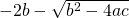if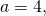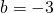and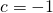.

For problems 2 and 3, solve for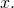1.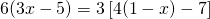2.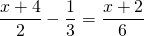3. Find the equation that has a slope of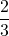and passes through the point (1, 4).
4. Find the distance between the points (−4, −2) and (4, 4).
5. Graph the relation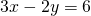.

For problems 7 and 8, find the solution set and graph it.

1.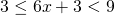2.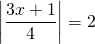In problems 9 and 10, set up each problem algebraically and solve. Be sure to state what your variables represent.

1. The weight (wm) of an object on Mars varies directly with its weight (we) on Earth. A person who weighs 95 lb on Earth weighs 38 lb on Mars. How much would a 240 lb person weigh on Mars?
2. Find two consecutive even integers such that their sum is 20 less than the second integer.

## Questions from Chapters 4 to 6

For problems 1–3, find the solution set of each system by any convenient method.

1.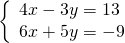2.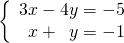3.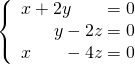For problems 4–6, perform the indicated operations and simplify.

1.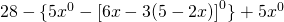2.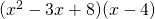3.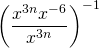For problems 7 and 8, factor each expression completely.

1.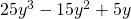2.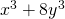3. How many litres of club soda (carbonated water) must be added to 2 litres of 35% fruit juice to turn it into a carbonated drink diluted to 8% fruit juice?
4. Kyra has 14 coins with a total value of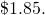If all the coins are dimes and quarters, how many of each kind of coin does she have?

## Questions from Chapters 7 to 9

In problems 1–3, perform the indicated operations and simplify.

1.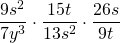2.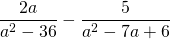3.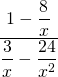For questions 4–6, simplify each expression.

1.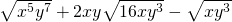2.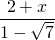3.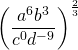For questions 7 and 8, solve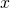by any convenient method.

1.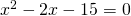2.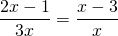In problems 9 and 10, find the solution set of each system by any convenient method.

1. The length of a rectangle is 5 cm longer than twice the width. If the area of the rectangle is 75 cm2, find its length and width.
2. Find three consecutive odd integers such that the product of the first and the second is 25 less than 8 times the third.

<a class=”internal” href=”/intermediatealgebraberg/back-matter/final-exam-version-b-answer-key/”>Final Exam: Version B